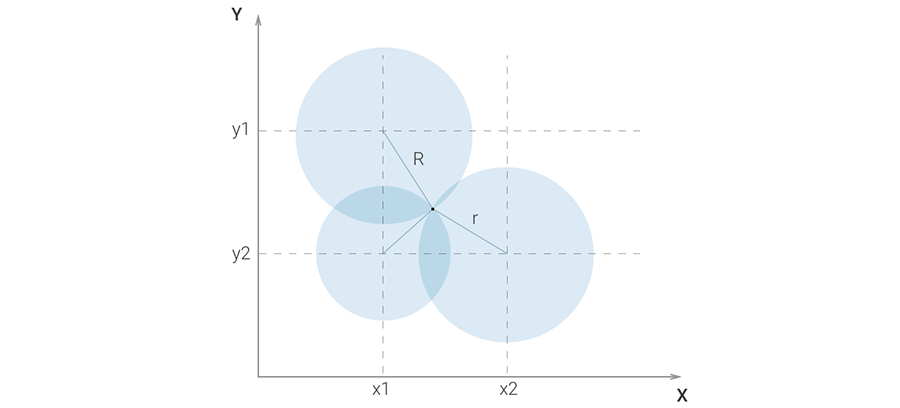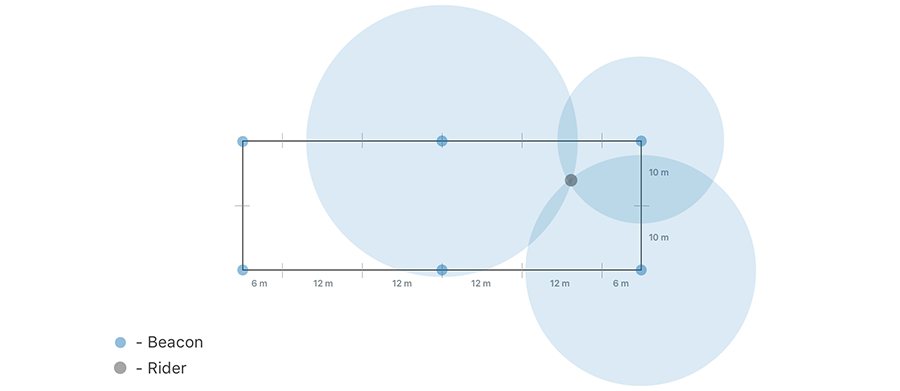3 minutes

# HorseAnalytics. Dressage. iBeacons Approach. Part 2

In this article we continue to explore if iBeacons can help to identify current position of a horse rider on the arena and to keep track of location change in a very accurate way. If you didn’t read the first part of the article - please follow the link.#### APPROACHThe problem of finding the user's position using the iBeacon comes down to finding the points of intersection of the three circles, the centers of which are the three nearest beacons and the radiuses are distances from the desired point.Points of circles' intersection are found by the following algorithm:

R - distance to the first Beacon, r - distance to the second Beacon. The positions of the first and the second Beacons - (x1, y1) and (x2, y2).

Let’s shift the beacon's position so the position of the first one is (0,0). Than the position of second will be (x2 - x1, y2 - y1)  = (a,b):

x+ y2 = R2

(x - a) 2 + (y - b) 2 = r 2

After opening parenthesis equations, we receive the following one:

x+ y2 = R2

x 2 + y 2 - 2ax - 2by + a 2 + b 2 = r 2

Now the first equation can be subtracted from the second one. Thus, squares in the variables disappear and there is a linear equation: - 2ax - 2by = r 2 - R 2 - a 2 - b 2.

With this equation it`s possible to express y through x: y = (r 2 - R 2 - a 2 - b 2 - 2ax) /2b.

If we substitute the found expression in the equation of the circle, the problem is reduced to solving a quadratic equation: mx 2 + px + q = 0, where

d = r 2 - R 2 - a 2 - b 2

m = 4*(a 2+ b 2)

p = 4*a*d,

q = - 4*b 2*R + d 2

The roots of this equation allow to find the coordinates of the intersection points of circles. To find coordinates of the points of intersection we need to add (x1, y1) in order to shift them to the real position. Since we received distance from the beacon to the device with error, it is unlikely that a common point of intersection of the three circles exists. So after finding the points of intersection for all pairs of circles, we found two points with the smallest distance between them and assume that the user will be located between these points.Since iBeacon returns integers, the maximum error for a distance of 20 m will be 2,2 mWe decided to use https://estimote.com ibeacons to check our assumptions. We will introduce 3 configurations of arena setup with iBeacons.

### Configuration A### Configuration B### Configuration CLooks like the approach is well described and the next step would be to check it. To be continued….Article Contents: